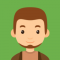## PHP bindec() FunctionPublished on 29-Jun-2020 08:32:59
Definition and UsageThe bindec() function returns decinmal equivalent of a binary number represented as a string argument. Binary number inside string is interpreted as unigned integer.This function returns a decimal integer. However, it may return float for size reasons.Syntaxbindec ( string \$binary_string ) : numberParametersSr.NoParameter & Description1binary_stringA string containing binary ... Read More

## PHP base_convert() FunctionPublished on 29-Jun-2020 08:30:58
Definition and UsageThe base_convert() function is versatile utility to convert a number with one base to another. The base is not restricted to binary, octal, hexadecimal or decimal. It can be any number between 2 and 36.First argument to this function is a string that can contain alpha-numeric characters. Digits ... Read More

## PHP atanh() FunctionPublished on 29-Jun-2020 08:28:34
Definition and UsageThe atanh() function returns the inverse hyperbolic tangent ratio of given given parameter. In other words, the return value of atanh() is hyperbolic tangent of given parameter. A inverse hyperbolic tangent function is defined as .atanh(x) = 0.5Xlog((1+x)/(1-x))This function returns a float value .Syntaxatanh ( float \$arg ) ... Read More

## PHP atan2() FunctionPublished on 29-Jun-2020 08:25:30
Definition and UsageThe atan2() function calculates arc tan of two variablesatan2(y, x) returns the arc tangent of the two numbers x and y. although it is similar to atan(y)/atan(x), the signs of both x and y are used to determine the quadrant of the result. Accordingly for values of x ... Read More

## PHP atan() FunctionPublished on 29-Jun-2020 08:23:31
Definition and UsageThe atan() function returns the arc tan or tan inverse of arg in radians. atan() is the inverse function of tan(). Therefore if tan(x)=y, atan(y)=x.For example, tan(pi/3)= 1.73205080757 (Squre Root of 3) and atan(1.73205080757)=1.04719755 rad which is equal to pi/3.This function returns a float value .Syntaxatan ( float ... Read More

## PHP asinh() FunctionPublished on 29-Jun-2020 08:21:53
Definition and UsageThe asinh() function calculates inverse of hyperbolic sine of given parameter. In other words, the return value of asinh() is hyperbolic sine of given parameter. A inverse hyperbolic sine function is defined asasinh(x) = log(x+sqrt(pow(x, 2)+1))This function returns a float value.Syntaxasinh( float \$arg ) : floatParametersSr.NoParameter & Description1argA ... Read More

## PHP acosh() FunctionPublished on 11-Jun-2020 08:38:01
Definition and UsageThe acosh() function returns the inverse hyperbolic cosine ratio of given angle in of given parameter. In other words, the return value of asinh() is hyperbolic sine of given parameter. A hyperbolic inverse hyperbolic cosine function is defined as −.acosh(x) = log(x+sqrt(pow(x, 2)-1))This function returns a float value.Syntaxacosh ... Read More

## PHP acos() FunctionPublished on 11-Jun-2020 08:32:50
Definition and UsageThe acos() function Returns the arc cosine or cos inverse of arg in radians. acos() is the inverse function of cos(). Therefore if cos(x)=y, acos(y)=x.For example, cos(pi/2)=0 and acos(0)=1.57079633 rad which is equal to pi/2.This function returns a float value.Syntaxacos ( float \$arg ) : floatParametersSr.NoParameter & Description1argA ... Read More

## PHP abs() FunctionPublished on 11-Jun-2020 08:25:30
Definition and UsageThe abs() function is an in-built function in PHP iterpreter. This function accepts any number as argument and returns a positive value, disregarding its sign. Absolute value of any number is always positive.This function always returns positive number.Syntaxabs( mixed \$num)ParametersSr.NoParameter & Description1numThis parameter stores a value whose absolute ... Read More